﻿ 基于AMF-HXA的湖南省城乡居民收入差异研究 Using AMF-HXA for Difference of Residents’ Income between Urban and Rural Areas of Hunan Province

Statistics and Application
Vol. 09  No. 01 ( 2020 ), Article ID: 33917 , 13 pages
10.12677/SA.2020.91004

Using AMF-HXA for Difference of Residents’ Income between Urban and Rural Areas of Hunan Province

Zewei Bai, Xinwei Wen, Shen Liu, Yazhi Li, Fang Wang*

College of Information and Intelligence, Hunan Agricultural University, Changsha Hunan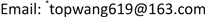Received: Dec. 20th, 2019; accepted: Jan. 6th, 2020; published: Jan. 13th, 2020ABSTRACT

With the rapid economic development of Hunan province, the per capita disposable income gap between urban and rural areas is becoming more and more obvious. The income imbalance not only reflects the difference of regional economic development, but also reflects the asynchronous development of urban and rural areas in the process of China’s reform and opening up. By using analogous multifractal height cross-correlation analysis (AMF-HXA), we study the per capita disposable income of difference and the cross correlation of the 14 cities in Hunan province. After that, by using the grey forecasting model, we forecast the income of urban and rural areas respectively. The results show that the fluctuation of per capita disposable income is small between cities and towns in developed areas, while that is large in poor areas. There is significant cross-correlation between the different regions we divided for both urban areas and rural areas. The fitting effect of grey prediction model is remarkable. These conclusions provide a new perspective for exploring the interaction between urban and rural per capita income in Hunan province.

Keywords:Analogous Multifractal Height Cross-Correlation Analysis, Per Capita Disposable Income, Grey Forecast1. 引言

2. 研究方法与数据来源

2.1. 基于AMF-HXA的q阶互相关系数 

$X\left(t\right)=\underset{i=1}{\overset{t}{\sum }}\left[{x}_{i}-〈x〉\right]\text{ }\text{ },Y\left(t\right)=\underset{i=1}{\overset{t}{\sum }}\left[{y}_{i}-〈y〉\right]\text{ }\text{ },$ (1)

${\Delta }_{L}X\left(t\right)Y\left(t\right)=\left[X\left(t\right)-X\left(t+L\right)\right]\cdot \left[Y\left(t\right)-Y\left(t+L\right)\right].$ (2)

${F}_{xy}^{q}\left(L\right)=\frac{1}{N-L}\underset{t=1}{\overset{N-L}{\sum }}\text{sgn}\left({\Delta }_{L}X\left(t\right)Y\left(t\right)\right){|{\Delta }_{L}X\left(t\right)Y\left(t\right)|}^{q/2},$ (3)

${\left[{F}_{xy}^{q}\left(L\right)\right]}^{1/q}={F}_{xy}\left(q,L\right)\propto {L}^{{\lambda }_{×}\left(q\right)}.$ (4)

${F}_{xx}\left(q,L\right)={〈{\Delta }_{L}X{\left(t\right)}^{q}〉}^{1/q}\propto {L}^{{\gamma }_{xx}}.$ (5)

$\Delta F\left(q,L\right)=\mathrm{log}{F}_{xx}\left(q,L\right)-\mathrm{log}{F}_{yy}\left(q,s\right),$ (6)

AMF-HXA方法能够准确检测两个时间序列的互相关性，然而，它不能定量地刻画这种互相关性。为了定量描述互相关的程度，基于AHXA (即取q = 2时)，利用公式(3)得到的两个序列的协方差函数又与公式(5)得到的两个序列的方差函数求比值，得到了一种所谓的基于AHXA的相关系数，如下所示：

$\rho \left(L\right)=\frac{{F}_{xy}^{2}\left(L\right)}{{F}_{xx}\left(2,L\right){F}_{yy}\left(2,L\right)}=\frac{〈\mathrm{sgn}\left({\Delta }_{L}X\left(t\right)Y\left(t\right)\right)|{\Delta }_{L}X\left(t\right)Y\left(t\right)|〉}{\sqrt{〈{\Delta }_{L}X{\left(t\right)}^{2}〉〈{\Delta }_{L}Y{\left(t\right)}^{2}〉}}.$ (7)

$\rho \left(L\right)$ 是一个无量纲量，且满足 $-1<\rho \left(L\right)<1$。为了刻画不同大小的波动区间对 $\rho \left(L\right)$ 的影响，我们对上述公式进行如下调整：

${\rho }_{q}\left(L\right)=\frac{{F}_{xy}^{q}\left(L\right)}{{F}_{xx}\left(q,L\right){F}_{yy}\left(q,L\right)}=\frac{{〈\mathrm{sgn}\left({\Delta }_{L}X\left(t\right)Y\left(t\right)\right)|{\Delta }_{L}X\left(t\right)Y\left(t\right)|〉}^{q/2}}{\sqrt{〈{\Delta }_{L}X{\left(t\right)}^{q}〉〈{\Delta }_{L}Y{\left(t\right)}^{q}〉}}.$ (8)

2.2. 灰色预测模型

${x}^{\left(1\right)}=\left({x}^{\left(1\right)}\left(1\right),{x}^{\left(1\right)}\left(2\right),\cdots ,{x}^{\left(1\right)}\left(n\right)\right)$，其中 ${x}^{\left(1\right)}\left(k\right)={\sum }_{i=1}^{k}{x}^{\left(0\right)}\left(i\right),k=1,2,\cdots ,n$。累加数列克服了原始数列的波动

$\frac{\text{d}{x}^{\left(1\right)}}{\text{d}t}+a{x}^{\left(1\right)}=b$ (9)

$\stackrel{^}{a}={\left[a,b\right]}^{\text{T}}={\left({B}^{\text{T}}B\right)}^{-1}{B}^{\text{T}}Y$ (10)

${\stackrel{^}{x}}^{\left(1\right)}\left(k+1\right)=\left({x}^{\left(0\right)}\left(1\right)-\frac{b}{a}\right){\text{e}}^{-ak}+\frac{b}{a},k=1,2,\cdots ,n,$ (11)

${\stackrel{^}{x}}^{\left(0\right)}\left(k+1\right)={\stackrel{^}{x}}^{\left(1\right)}\left(k+1\right)-{\stackrel{^}{x}}^{\left(1\right)}\left(k\right),k=1,2,\cdots ,n.$ (12)

$q\left(k\right)={x}^{\left(0\right)}\left(k\right)-{\stackrel{^}{x}}^{\left(0\right)}\left(k\right),$ (13)

$\epsilon \left(k\right)=\frac{q\left(k\right)}{{x}^{\left(0\right)}\left(k\right)}×100%=\frac{{x}^{\left(0\right)}\left(k\right)-{\stackrel{^}{x}}^{\left(0\right)}\left(k\right)}{{x}^{\left(0\right)}\left(k\right)}×100%.$ (14)

$\epsilon \left(avg\right)=\frac{1}{n-1}\underset{k=2}{\overset{n}{\sum }}|\epsilon \left(k\right)|\text{ }\text{ }.$ (15)

${p}^{0}=\left(1-\epsilon \left(avg\right)\right)×100%.$ (16)

2.3. 数据来源

3. 互相关性检测与分析

3.1. 互相关波动函数幂律关系检测Table 1. Basic statistics of per capita disposable income in various regions of Hunan Province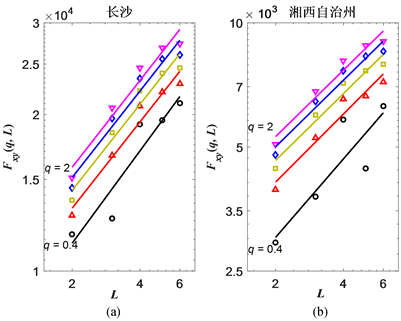Figure 1. Double-log plot of ${F}_{xy}\left(q,L\right)$ and L of Changsha (a) and Xiangxi (b)

3.2. 波动差异与时滞的关系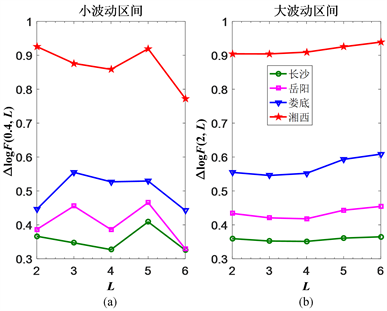Figure 2. $\Delta F\left(q,L\right)$ of small and large fluctuation intervals in Changsha, Xiangxi, Loudi and Zhuzhou

3.3. 各市州及区域人均收入差异的相关分析

3.3.1. 各市州农村与城镇人均收入的相关性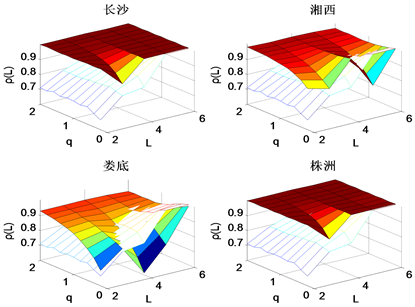Figure 3. Cross-correlated coefficient between urban and rural income in Changsha, Loudi, Zhuzhou and Xiangxi. Hollow surface denotes critical valuesTable 3. ρq(L) (q = 2) values of large fluctuation intervals in 14 cities

3.3.2. 各城市群之间人均收入的相关性

4. 灰色预测结果Table 4. The average relative error between the predicted and observed values of per capita income in each cities of Hunan Province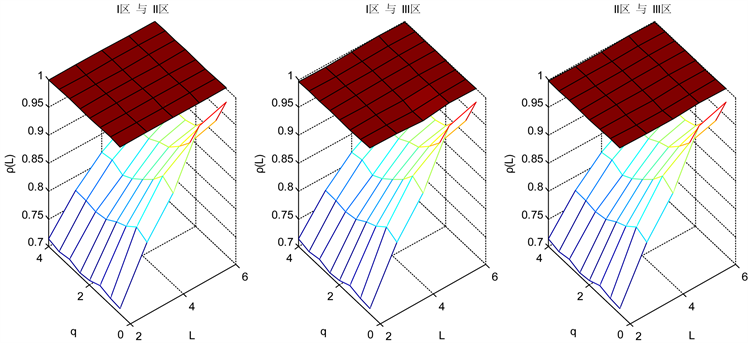Figure 4. Cross-correlated coefficient of urban income between Region I and Region II, Region I and Region III, Region II and Region III, respectively. Hollow surface denotes critical values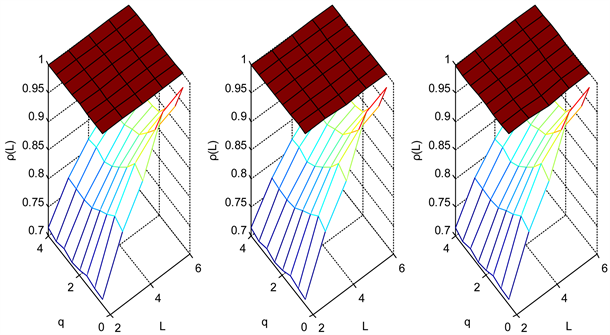Figure 5. Cross-correlated coefficient of rural income between Region I and Region II, Region I and Region III, Region II and Region III, respectively. Hollow surface denotes critical values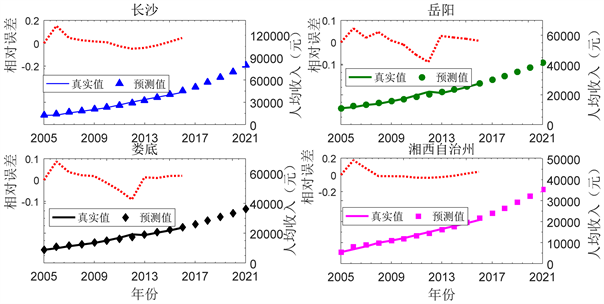Figure 6. The forecast effect of urban per capita income in Changsha, Yueyang, Loudi, and Xiangxi, respectively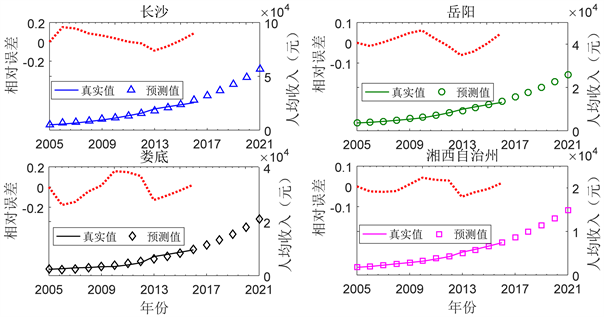Figure 7. The forecast effect of rural per capita income in Changsha, Yueyang, Loudi, and Xiangxi, respectively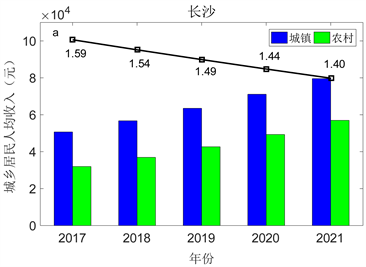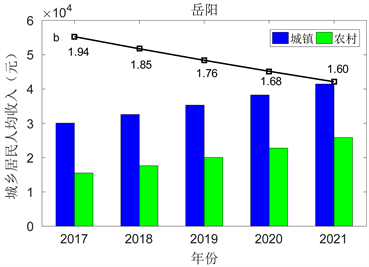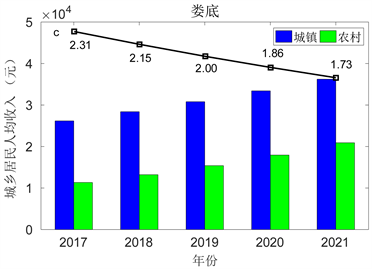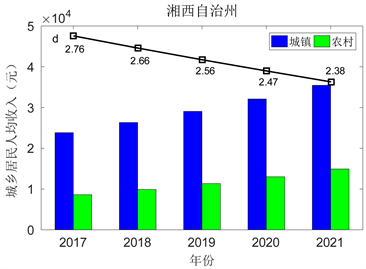Figure 8. Urban and rural disposable per capita income of Changsha, Loudi and Xiangxi in the years of 2017-2021

5. 结语

1) 14个市州的城乡人均收入的q阶互相关波动函数 ${F}_{xy}\left(q,L\right)$ 与时间间隔L均具有良好的幂律关系，说明城镇与乡村之间的人均收入具有显著的互相关；2) 通过对波动差距函数的研究发现，经济水平越高的地区其城镇与农村的波动函数差距都越小；3) 通过对14个市州的城乡人均收入的 ${\rho }_{q}\left(L\right)$ 在不同波动范围内的研究发现：大波动范围内各个市州农村与城镇的人均收入具有高度互相关性；4) 通过对所划分城市群的研究发现，湖南省各个城市群间具有显著的互相关性。5) 运用GM(1,1)灰色预测模型对过去12年人均收入数据进行拟合建模，模型精度为优秀，并预测未来5年的湖南省各市州的人均收入，城乡差距逐年缩小。

Using AMF-HXA for Difference of Residents’ Income between Urban and Rural Areas of Hunan Province[J]. 统计学与应用, 2020, 09(01): 26-38. https://doi.org/10.12677/SA.2020.91004

1. 1. 刘文, 房光婷. 珠三角、长三角、环渤海区域城乡居民收入差距研究[J]. 云南财经大学学报, 2010(1): 132-143.

2. 2. 景跃军, 李雪. 我过城乡居民收入区域差异分析与对策[J]. 经济与管理, 2014, 28(2): 34-38.

3. 3. Bahmani-Oskooee, M. and Motavallizadeh-Ardakani, A. (2018) On the Effects of Income Volatility on Income Distribution: A Symmetric Evidence from State Level Data in the US. Research in Economics, 72, 224-239. https://doi.org/10.1016/j.rie.2018.01.002

4. 4. Yang, L.M., Kong, L.L., Shen, Y.N. and Ge, Y.Y. (2014) Analysis on Regional Difference in Relationship between Financial Development and Urban-Rural Income Gap. In: Proceedings of the Eighth International Conference on Management Science and Engineering Management, Springer, Berlin, Heidelberg, 325-335. https://doi.org/10.1007/978-3-642-55182-6_29

5. 5. Ma, X., Wang, F.R., Chen, J.D. and Zhang, Y. (2017) The Income Gap between Urban and Rural Residents in China: Since 1978. Computational Economics, 52, 1153-1174. https://doi.org/10.1007/s10614-017-9759-4

6. 6. 彭真善. 中国东、中、西部地区城乡收入差距比较分析[J]. 经济地理, 2009, 29(7): 1087-1091.

7. 7. 王少国. 我国城乡收入差距的地区类型分析[J]. 技术经济与管理研究, 2011(5): 85-88.

8. 8. Podobnik, B. and Stanley, H.E. (2008) Detrended Cross-Correlation Analysis: A New Method for Analyzing Two Nonstationary Time Series. Physical Review Letters, 100, 84-102. https://doi.org/10.1103/PhysRevLett.100.084102

9. 9. Zebende, G.F. (2011) DCCA Cross-Correlation Coefficient: Quantifying Level of Cross-Correlation. Physica A, 390, 614-618. https://doi.org/10.1016/j.physa.2010.10.022

10. 10. Zhou, W.X. (2008) Multifractal Detrended Cross-Correlation Analysis for Two Nonstationary Signals. Physical Review E, 77, Article ID: 066211. https://doi.org/10.1103/PhysRevE.77.066211

11. 11. Kalamaras, N., Philippopoulos, K. and Deligiorgi, D. (2017) Scaling Properties of Meteorological Time Series Using Detrended Fluctuation Analysis. Perspectives on Atmospheric Sciences, 78, 545-550. https://doi.org/10.1007/978-3-319-35095-0_78

12. 12. Yin, Y. and Shang, P.J. (2015) Multiscale Multifractal Detrended Cross-Correlation Analysis of Traffic Flow. Nonlinear Dynamics, 81, 1329-1347. https://doi.org/10.1007/s11071-015-2072-7

13. 13. Wang, F., Liao, G.P., Li, J.H., Zou, R.B. and Shi, W. (2013) Cross-Correlation Detection and Analysis for California’s Electricity Market Based on Analogous Multifractal Analysis. Chaos, 23, Article ID: 013129. https://doi.org/10.1063/1.4793355

14. 14. Wang, F., Yang, Z.H. and Wang, L. (2016) Detecting and Quantifying Cross-Correlations by Analogous Multifractal Height Cross-Correlation Analysis. Physica A, 444, 954-962. https://doi.org/10.1016/j.physa.2015.10.096

15. 15. Wang, F., Wang, L. and Chen, Y.M. (2018) Quantifying the Range of Cross-Correlated Fluctuations Using a q-L Dependent AHXA Coefficient. Physica A, 494, 454-464. https://doi.org/10.1016/j.physa.2017.12.009

16. 16. Barabási, A.L., Szépfalusy, P. and Vicsek, T. (1991) Multifractal Spectra of Multi-Affine Functions. Physica A, 178, 17-28. https://doi.org/10.1016/0378-4371(91)90072-K

17. 17. 段杰, 张娟. 基于灰色预测的深圳文化创意产业发展对经济增长贡献研究[J]. 中国人口•资源与环境, 2014, 24(S1): 457-460.

18. 18. Lu, P., Cai, S., Yang, P. and Rosenbaum, M.S. (2005) Disintegration Characteristics of Weak Rocks Using the Grey Prediction Technique. Geotechnical & Geological Engineering, 23, 131-139. https://doi.org/10.1007/s10706-003-5969-8

19. 19. 赵楠. 经济变量间满足幂律关系的一些研究[D]: [硕士学位论文]. 武汉: 华中科技大学, 2013.

20. 20. 郑烨, 王春萍, 张顺翔, 李子恒. 精准扶贫提升农户满意度的作用机制研究——基于西部某省三贫困县的实证调查[J]. 软科学, 2018(11): 15-19.

NOTES

*通讯作者。